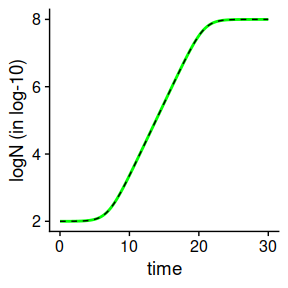# About the units of the growth rate (mu)

library(biogrowth)
library(tidyverse)
library(cowplot)

One of the aspects that often lead to confusion in growth modeling are the units of the parameter describing the maximum growth rate ($$\mu$$). This is due to the fact that, although the parameter has units of $$[TIME]^{-1}$$, the scale of the logarithm of the population size introduces a multiplying constant in this parameter. By default, biogrowth makes all the calculations using log10 scale. However, the fit_growth() and predict_growth() functions include the logbase_mu argument to accommodate for other units systems.

This vignette tries to clarify this point about the units for $$mu$$ under both isothermal and dynamic conditions. It also illustrates how the logbase_mu argument should be used.

## Growth rate under constant environmental conditions

### Mathematical justification

Under constant environmental conditions, the typical growth curve has a sigmoidal shape:

#> Scale for 'y' is already present. Adding another scale for 'y', which will
#> replace the existing scale.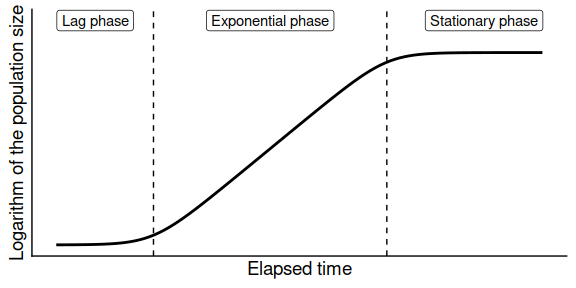Then, parameter $$\mu$$ is defined as the slope of growth curve during the exponential phase. This can be expressed as

$\mu = \frac{\log N_{i+\Delta} - \log N_i}{\Delta t}$

where $$\log N_i$$ and $$\log N_{i+\Delta}$$ are the population sizes at two time points separated a distance $$\Delta t$$. Because $$\log N$$ is unitless, $$\mu$$ has units of $$[TIME]^{-1}$$. However, the base of the logarithm affects the value of $$\mu$$.

As an example, let us assume that the exponential growth phase starts with a concentration $$N=1$$. Let’s also assume that after 10 hours, the population has increased to $$N=100$$ (while remaning in the exponential phase). If we make the calculations of the growth rate in natural ($$e$$) scale, we would calculate a value of $$\mu$$ of:

$\mu_e = \frac{\ln 100 - \ln 1}{10} = \frac{4.6 - 0}{10} = 0.46 \space h^{-1}$

Whereas, if we do the calculations using base 10 for the logarithms, we would calculate a value of $$\mu$$ of:

$\mu_{10} = \frac{\log_{10} 100 - \log_{10} 1}{10} = \frac{2 - 0}{10} = 0.2 \space h^{-1}$

Therefore, although $$\mu$$ has units of $$[TIME]^{-1}$$, the scale of the logarithm of the population size affects its value. For this reason, it is advisable to include the base of the logarithm in the units of $$\mu$$ (such as $$\mu = 0.46 \ln CFU/h$$ or $$\mu = 0.2 \log_{10} CFU/h$$).

The conversion between both unit systems is very simple, just requiring the multiplication by a change of base:

$\mu_b = \mu_a \cdot \log_b a$

So, a growth rate expressed in log10 scale can be converted to natural scale by

$\mu_{e} = \mu_{10} \cdot \ln 10 = 0.2 \cdot 2.3 = 0.46 \ln CFU/h$

### Implementation in biogrowth

The function predict_growth() includes the logbase_mu argument to account for different units of $$\mu$$. By default, all the calculations are done in log10 scale. Therefore, the value of $$mu$$ indicates the time required for one log-increase in the exponential phase. This is illustrated in this plot, where the time for 1 log(10) increase in the exponential growth phase is defined by $$\mu$$ (mu=1).

predict_growth(seq(0, 5, length = 100),
list(model = "Trilinear",
logN0 = 2, lambda = 1, logNmax = 9, mu = 1),
environment = "constant") %>%
plot() +
theme_gray()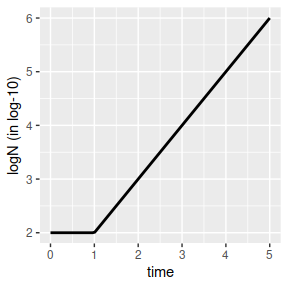Instead of the default log10 base, $$\mu$$ can be defined in other units using the logbase_mu argument. For instance, this parameter can be defined in natural scale (i.e. $$e=e^1=\exp (1)$$) passing logbase_mu=exp(1).

predict_growth(seq(0, 5, length = 100),
list(model = "Trilinear",
logN0 = 2, lambda = 1, logNmax = 9, mu = 1),
environment = "constant",
logbase_mu = exp(1)) %>%
plot() +
theme_gray() +
ylim(2, 6)
#> Scale for 'y' is already present. Adding another scale for 'y', which will
#> replace the existing scale.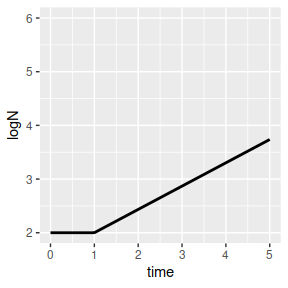Note that, in this case, $$mu$$ no longer corresponds to the slope of the growth curve because the y-axis is defined in a different scale.

The following plot shows how using the right conversion ($$\mu_b = \mu_a \cdot \log_b a$$) results in equivalent growth curves under isothermal conditions:


my_time <- seq(0, 20, length = 1000)  # Vector of time points for the calculations

list(
base 2 = predict_growth(my_time,
list(model = "Baranyi",
logN0 = 0, logNmax = 6, mu = 1*log(10, base = 2),
lambda = 5),
environment = "constant",
logbase_mu = 2),
base 10 = predict_growth(my_time,
list(model = "Baranyi",
logN0 = 0, logNmax = 6, mu = 1,
lambda = 5),
environment = "constant"),
base e = predict_growth(my_time,
list(model = "Baranyi",
logN0 = 0, logNmax = 6, mu = 1*log(10),
lambda = 5),
environment = "constant",
logbase_mu = exp(1))
) %>%
map(., ~.$simulation) %>% imap_dfr(., ~ mutate(.x, model = as.character(.y))) %>% ggplot() + geom_line(aes(x = time, y = logN, colour = model, linetype = model, size = model)) + scale_size_manual(values = c(3, 2, 1))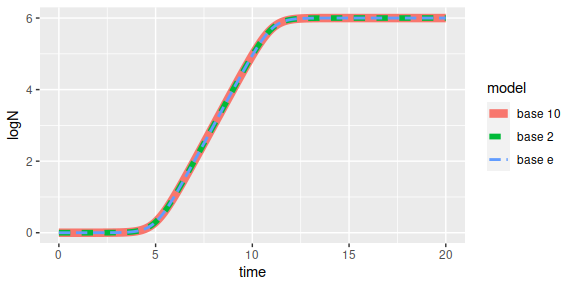### Unit interpration for model fitting The same considerations apply to fitting a model to data gathered under constant environmental conditions. By default, the model is fitted using log10 scale for mu. my_data <- data.frame(time = c(0, 25, 50, 75, 100), logN = c(2, 2.5, 7, 8, 8)) models <- list(primary = "Baranyi") known <- c(lambda = 25) start <- c(logNmax = 8, logN0 = 2, mu = .3) primary_fit10 <- fit_growth(my_data, models, start, known, environment = "constant" ) This is represented in the print method for the instance of FitIsoGrowth primary_fit10 #> Primary growth model fitted to data #> #> Growth model: Baranyi #> #> Estimated parameters: #> logNmax logN0 mu #> 8.0000221 2.0994862 0.1978506 #> #> Fixed parameters: #> lambda #> 25 #> #> Parameter mu defined in log-10 scale #> Population size defined in log-10 scale and is saved as the logbase_mu argument primary_fit10$logbase_mu
#>  10

This base can be changed using the logbase_mu argument. For instance, we can set it to natural scale (logbase_mu = exp(1)):

primary_fit_e <- fit_growth(my_data, models, start, known,
environment = "constant",
logbase_mu = exp(1)
)

Note that, in both cases, the models fitted are identical

plot_grid(plot(primary_fit_e), plot(primary_fit10))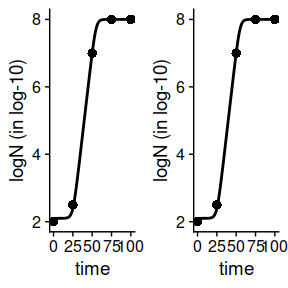However, the parameter estimates for $$\mu$$ are different due to the use of a different scale

print(primary_fit_e)
#> Primary growth model fitted to data
#>
#> Growth model: Baranyi
#>
#> Estimated parameters:
#>   logNmax     logN0        mu
#> 8.0000221 2.0994862 0.4555678
#>
#> Fixed parameters:
#> lambda
#>     25
#>
#> Parameter mu defined in log-e scale
#> Population size defined in log-10 scale
print(primary_fit10)
#> Primary growth model fitted to data
#>
#> Growth model: Baranyi
#>
#> Estimated parameters:
#>   logNmax     logN0        mu
#> 8.0000221 2.0994862 0.1978506
#>
#> Fixed parameters:
#> lambda
#>     25
#>
#> Parameter mu defined in log-10 scale
#> Population size defined in log-10 scale

Both parameter values can be converted using the usual transformation

coef(primary_fit10)["mu"] * log(10)
#>        mu
#> 0.4555678

## Dynamic environmental conditions

Under dynamic environmental conditions, exponential growth is often described as a first order differential equation:

$\frac{dN}{dt} = \mu \cdot N$

In this case, the growth rate is defined in natural scale (i.e. base $$e$$). Nonetheless, the model can be easily adapted to any base ($$b$$) using the equation described above:

$\frac{dN}{dt} = \mu_b \cdot \ln b \cdot N$

When making predictions, this definition has the same issues in the interpretation as the previous case. As an example, let us define a model defined based on secondary models (with large $$Q_0$$ to avoid a lag phase)

q0 <- 1e5
mu_opt <- 1  # in log10 scale

my_primary <- list(mu_opt = mu_opt,
Nmax = 1e8,N0 = 1e2,
Q0 = q0)

sec_temperature <- list(model = "CPM",
xmin = 5, xopt = 35, xmax = 40, n = 2)

my_secondary <- list(temperature = sec_temperature)

If we make a prediction for a profile with constant temperature:

my_conditions <- data.frame(time = c(0, 5),
temperature = c(35, 35)
)

my_times <- seq(0, 5, length = 1000)

dynamic_prediction <- predict_growth(environment = "dynamic",
my_times,
my_primary,
my_secondary,
my_conditions)

Because, by default, the calculations are done in log10 scale, the value of $$\mu$$ (mu_opt=1) still matches the time required to increase the population size in one log10:

plot(dynamic_prediction) + theme_gray()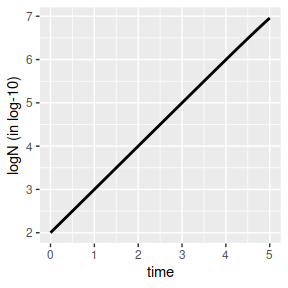However, if the calculations are done using natural base for $$\mu$$, this is not the case any more.

predict_growth(environment = "dynamic",
my_times,
my_primary,
my_secondary,
my_conditions,
logbase_mu = exp(1)) %>%
plot() + theme_gray() + ylim(2, 7)
#> Scale for 'y' is already present. Adding another scale for 'y', which will
#> replace the existing scale.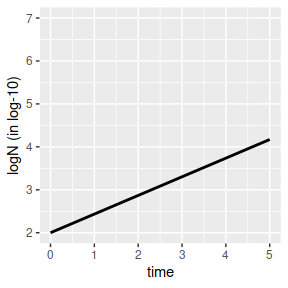For the same reasons as for the static case, we must use the unit conversion of the growth rate to obtain equivalent model predictions:


my_conditions <- data.frame(time = c(0, 5, 40),
temperature = c(20, 30, 35)
)

sec_temperature <- list(model = "Zwietering",
xmin = 25, xopt = 35, n = 1)

my_secondary <- list(
temperature = sec_temperature
)

my_times <- seq(0, 50, length = 1000)

list(base 10 = predict_growth(environment = "dynamic",
my_times,
list(mu = 2, Nmax = 1e7, N0 = 1, Q0 = 1e-3),
my_secondary,
my_conditions
),
base e = predict_growth(environment = "dynamic",
my_times,
list(mu = 2*log(10), Nmax = 1e7, N0 = 1, Q0 = 1e-3),
my_secondary,
my_conditions,
logbase_mu = exp(1)
),
base 2 = predict_growth(environment = "dynamic",
my_times,
list(mu = 2*log(10, base=2), Nmax = 1e7, N0 = 1, Q0 = 1e-3),
my_secondary,
my_conditions,
logbase_mu = 2
)
) %>%
map(., ~.$simulation) %>% imap_dfr(., ~ mutate(.x, model = as.character(.y))) %>% ggplot() + geom_line(aes(x = time, y = logN, colour = model, linetype = model, size = model)) + scale_size_manual(values = c(3, 2, 1))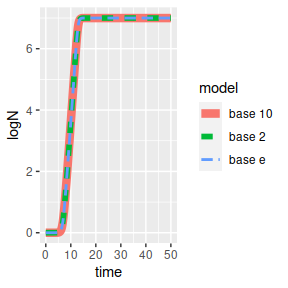## A note when comparing predictions based on primary models against models with secondary models As described in the vignette Using models based on secondary models to predict growth under constant environmental conditions, in some situations it can be advantageous the use of models based on secondary models to make predictions under static environmental conditions. However, one must be very careful with the unit system when making these predictions as the relationship between $$\lambda$$ and $$Q_0$$ is also affected by the units system. As an illustration, let’s define a growth model based on secondary models. q0 <- 1e-3 mu_opt <- 1 # in ln scale my_primary <- list(mu_opt = mu_opt, Nmax = 1e8, N0 = 1e2, Q0 = q0) sec_temperature <- list(model = "CPM", xmin = 5, xopt = 35, xmax = 40, n = 2) my_secondary <- list(temperature = sec_temperature) my_conditions <- data.frame(time = c(0, 30), temperature = c(35, 35) ) my_times <- seq(0, 30, length = 1000) dynamic_prediction <- predict_growth(environment = "dynamic", my_times, my_primary, my_secondary, my_conditions, logbase_mu = exp(1)) plot(dynamic_prediction)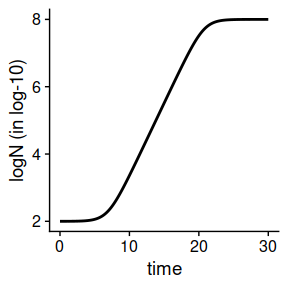In the Baranyi model, the parameter $$Q_0$$ is related to the duration of the lag phase under static conditions by $\lambda = \frac{ \log (1 + 1/Q_0 )}{\mu}$ However, this relationship is also affected by the same issues with the base of the logarithm. If this is not accounted for when making predictions under static conditions (i.e. based on a primary model that defines $$\lambda$$), there will be a discrepancy between the models. bad_lambda <- Q0_to_lambda(q0, mu_opt) bad_primary_model <- list(model = "Baranyi", logN0 = 2, logNmax = 8, mu = mu_opt, lambda = bad_lambda) bad_prediction <- predict_growth(my_times, bad_primary_model, logbase_mu = exp(1)) plot(bad_prediction, line_col = "red") + geom_line(aes(x = time, y = logN), linetype = 2, data = dynamic_prediction$simulation)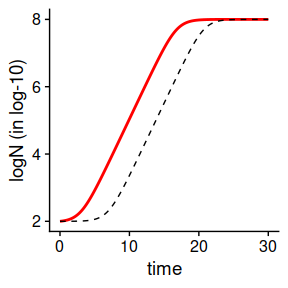In order to propertly compare between both modelling approaches, the argument logbase_mu has to be defined when calling Q0_to_lambda (or, equivalently, lambda_to_Q0).

good_lambda <- Q0_to_lambda(q0, mu_opt, logbase_mu = exp(1))

good_primary_model <- list(model = "Baranyi",
logN0 = 2, logNmax = 8, mu = mu_opt,
lambda = good_lambda)

good_prediction <- predict_growth(my_times, good_primary_model,
logbase_mu = exp(1))

plot(good_prediction, line_col = "green") +
geom_line(aes(x = time, y = logN), linetype = 2,
data = dynamic_prediction\$simulation)